﻿ Power Flow Calculation of Three-terminal DC Grid Considering Power Flow ControllerPublications are Open
Access in this journal
Article Versions
Export Article
• Normal Style
• MLA Style
• APA Style
• Chicago Style
Research Article
Open Access Peer-reviewed

### Power Flow Calculation of Three-terminal DC Grid Considering Power Flow Controller

Xunyu Liu , Xiaotian Xu, Yu Qi Pang, Xinyuan Zhang
American Journal of Electrical and Electronic Engineering. 2020, 8(3), 73-79. DOI: 10.12691/ajeee-8-3-1
Received June 18, 2020; Revised July 19, 2020; Accepted July 29, 2020

### Abstract

In terms of large-scale integration of renewable energy into the grid, DC grid technology has obvious advantages over traditional AC grids. The good operation of a DC grid depends on effective control of power flow distribution. However, the adjustment of the bus voltage and its total power by the converter station alone cannot guarantee the control of all branches of the power grid. Therefore, it is necessary to install a DC power flow controller to achieve a reasonable distribution of line flow. This article is aimed at the multi-terminal DC grid, based on the basic power flow calculation method, considers the influence of the power flow controller on the DC grid power flow calculation and adds the influence to the calculation, changing the power equation or resistance parameters of the line, thereby realizing the power flow calculation The grid power flow calculation of the controller is realized by programming in MATLAB. The proposed calculation method can lay the foundation for the power flow optimization and stable operation of the DC grid.

### 1. Introduction

In recent years, with the increasingly serious problems of fossil fuel consumption and environmental pressure, China and even the whole world are facing energy problems, and the future renewable energy will become the main force of primary energy 1. Wind energy, solar energy, ocean energy, etc., are all clean energy with intermittent and random characteristics. Therefore, the access network of this large-scale renewable energy puts forward a higher level of traditional power equipment, grid structure, and operation technology requirements. The traditional AC power grid only relies on AC circuit breakers to adjust the power flow of the grid, which can no longer meet the requirements for the response speed of the grid after the renewable energy is connected to the grid. Therefore, in order to respond to changes in the future energy landscape, it is imperative to develop new technologies, equipment and grid structures. The DC power grid has obvious advantages in some aspects. Compared with the AC power grid, it can accept large-scale and fluctuating new energy sources and improve the quality of power supply. The DC grid is an energy transmission system composed of a large number of DC terminals interconnected in the form of DC. It can smoothly access new energy, independently control active and reactive power, more flexible power supply mode and safe power flow control 2. Therefore, the establishment of a DC grid is an important development direction to realize large-scale access to renewable energy in the grid 3.

In this regard, scholars proposed to use DC grid to solve this problem, which is a hot topic of discussion among many solutions 4. Reference 5 founds a DC power grid with different voltage levels. After multiple wind turbines are rectified and output, they are collected on a common DC bus. After being commutated by a converter, a high-voltage DC transmission is finally formed. Finally, in this way Feed into the AC grid. Reference 6 proposes to adjust the power flow by changing the line parameters, that is, to connect variable resistors in series in the line, and adjust the branch power flow through the input and removal of the resistors. The variable resistance is controlled according to the different types of access switches. The devices are divided into mechanical switch series and semiconductor switch series. As a comparison, a DC converter is also proposed as a power flow controller to clarify its control characteristics. Reference 7 introduced three different power flow controllers: DC transformer, variable resistor, and series voltage source, and compared the control characteristics (control range, rated working condition, power loss) of the three controllers. Reference 8 proposed a DC power flow controller composed of back-to-back six-pulse converter bridges controlled by two thyristors, which has the characteristics of two-way power flow control and power four-quadrant operation.

This paper summarizes and classifies the existing DC power flow controllers, equates the external characteristics of various types of power flow controllers, and analyzes the influence of different types of controllers on the power flow of the DC system. Next, this article uses the traditional Newton-Raphson power flow calculation method for DC power flow calculation through the analogy of AC and DC systems 9, 10, 11, and then adds the influence of various types of controllers to In the power flow calculation, the DC grid power flow calculation with a power flow controller is realized. The proposed calculation method is realized by programming in MATLAB. Finally, the method is applied to a three-terminal DC grid equipped with a power flow controller. The calculation results are compared with the calculation results of MATLAB power flow analysis software PSAT to verify the proposed method. The correctness of the method.

### 2. DC Grid Power Flow Calculation

The construction of DC power grids around the world does not start from scratch, an independent power grid, which relies on the existing AC power grid. According to research, there are four main methods for calculating the basic power flow of traditional AC power grids, namely, Newton-Raphson iterative method, Gaussian iterative method based on admittance matrix, fast decomposition method, and algorithm based on impedance matrix. The advantage of the Gaussian method is that the memory is small, but the convergence is poor; the algorithm based on the impedance matrix has the advantage of good convergence, but it takes up a lot of memory. In the power flow calculation of the power system, its essence is to solve a set of nonlinear equations represented by the power equation, and the New pull method is an effective method to solve the nonlinear algebraic equations, so the New pull method is widely used in power flow. In calculation 12; the fast decomposition method is an improved Niu La method, and its calculation speed is greatly improved. Therefore, the analysis of the power flow and optimal power flow of the DC power grid is based on the AC/DC hybrid system formed by the AC power grid connected to it. Therefore, this section mainly analyzes the DC grid power flow calculation in the AC/DC hybrid system.

2.1. Newton-Raphson Method

The power flow calculation of the traditional AC system only needs four state variables of the node: voltage amplitude, voltage phase angle, active power, and reactive power. The four state quantities can be divided into four types of nodes: PQ node, PV node, and balance node. For the DC system, the nodes have only two quantities: active power and voltage level, so there are only two nodes, P and V. The P node indicates that the input active power of the node is known, and the V node indicates that the voltage of the node is known.

To sum up, the DC system power flow calculation process is to select a node from the system nodes. This node is the V node with a known voltage. The rest of the nodes naturally become P nodes. Then the solved state variable is the voltage of the P node. The calculation process of the DC power grid power flow is derived as shown in Figure 1.

• Figure 1. Flow chart of power flow calculation of Nullafa DC grid

We know that there is only resistance in the DC grid, so in the calculation process, the node parameter matrix is the node conductance matrix G. The active power calculation expression of node i is: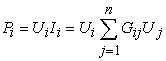(1)

Among them, n is the number of nodes, Ui is the voltage of node i, Ii is the current of node i, Gii is the self-conductance of node i, and Gij is the mutual conductance between nodes i and j. Thus, the Jacobian matrix structure is: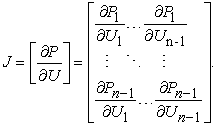(2)

Assuming that the nth node is a V node, the Jacobian matrix elements are calculated: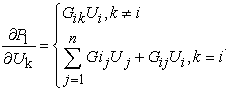(3)
2.2. Fast Decomposition

In the Newton Raphson method, the Jacobian matrix is a variable function that needs to be solved. After each iteration, a new Jacobian matrix is generated, and then the correction equation needs to be recalculated to obtain a new correction. This leads to each iteration. A lot of calculations. In order to reduce the amount of calculation, scholars proposed to use a definite Jacobian matrix instead of a variable Jacobian matrix to avoid repeated calculations. In 1974, scholar Stott discovered a fast decomposition method, which is widely used in AC power flow calculation.

The formula (2) is abbreviated as follows 12: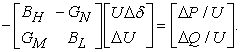(4)

In the formula, the elements in the Jacobian matrix are represented by BH, GN, GM, BL. We know that the voltage amplitude and phase angle affect the reactive power and active power of the power system respectively, so we can set the value of G to 0 to achieve decoupling of active and reactive power flow. In addition, scholar Sotto found through a lot of practice that the diagonal blocks BH and BL can be simplified into constant matrices, and there is a certain relationship between the constant matrices and the branch admittance: for the matrix BH to form a node conduction that ignores the influence of the ground branch capacitance and branch resistance. Nano matrix B', and matrix BL forms the imaginary part matrix B” after removing the PV node, and the voltage amplitude in UΔδ takes the unit value 1. The simplified correction equation is thus obtained: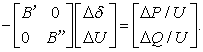(5)

The main purpose of the fast decomposition method is to simplify the Jacobian matrix into a constant matrix, which solves the problem of recalculating each iteration, and can get the same result as the Niula method. Although the number of iterations has increased, the amount of iterative calculation is very small, so in general, the calculation amount is smaller than that of the Niula method. Therefore, the fast decomposition method is particularly suitable for calculating the power flow of large-scale networks.

2.3. Optimal Power Flow of DC Grid

The optimal power flow of the power system is defined as: the system load has certain structural parameters. Under this condition, the control variables meet all the constraint conditions, and certain indicators of the system reach the best trend distribution. The optimal power flow algorithm proposed in this paper is to determine the voltage reference value through sensitivity analysis.

Analyze the sensitivity relationship between the voltage changes at different nodes, and extend the V node to equation (4), we can get: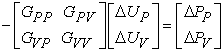(6)

When the voltage of the P node changes, its input power remains unchanged, which can be derived: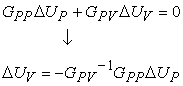(7)

Sensitivity analysis method: firstly analyze the voltage changes of the P and V nodes to determine the change of the P node, and finally solve the V node voltage-the optimal voltage reference value based on the sensitivity relationship.

Therefore, the optimal power flow calculation process is shown in Figure 2.

• Figure 2. Optimal power flow calculation process

### 3. DC Power Flow Controller

In the known DC transmission system, the output power flow at a certain end of the line is determined by the line resistance, the node voltage value, and the voltage drop across the line. When the line resistance and node voltage in the DC system change, the power flow of the system will also change. The controller changes the line resistance, which is the resistance type, and the controller changes the node voltage, which is the voltage type. The working principle and external characteristics of these two controllers are quite different, which will be introduced one by one below.

3.1. Variable Resistance Type
3.1.1. Working Principle

The variable resistance type realizes power flow control by changing the resistance of the line by inserting a resistance in the DC line. Its topology is shown in Figure 3. The resistors R1-Rn are connected in series, and the line resistance is changed by turning off the switches S1-Sn. In the existing variable resistance controller, the switches S1-Sn can be semiconductor or mechanical switches.

• Figure 3. Variable resistance type power flow controller topology

The advantage of this controller is that the structure and control are relatively simple, but this controller adds a resistor, which is a positive element, because it can only reduce the line current in the original power flow distribution and cannot change the direction of the power flow. In addition, the resistors connected in series are consumables, so they are generally not used in actual situations.

3.1.2. External Characteristics

It can be seen from the analysis of 3.1.1 that the essence of the variable resistance controller is to connect a resistor that can adjust the resistance to the line, and its external characteristics can be equivalent to a variable resistance. The simplified model is shown in Figure 4.

• Figure 4. External characteristics of variable resistance type power flow controller

According to the equivalent, the effect of the controller on the system is to change the resistance of its branches. Assuming that the resistance of the original line is R12, the equivalent line resistance after adding the controller becomes R12+RX. We turn the plus sign relationship into a multiple relationship so that we can analyze the controller's influence on the system more clearly. Therefore, the equivalent resistance after adding the controller can be expressed as R12: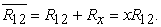(8)
3.2. Variable Voltage Type

The DC power flow controllers we are familiar with, such as DC/DC converters, thyristor-controlled power flow controllers, IGBT-controlled series voltage controllers 13, modular multi-level DC converters and current power flow controllers 14, are mostly Variable voltage type.

3.2.1. Working Principle

According to the different installation methods of the controller, it can be divided into parallel type and series type, and they change the voltage in different ways. The topology is shown in Figure 5.

• Figure 5. Variable voltage type power flow controller topology

After the parallel controller is added to the line, the port voltage U1 of the line is changed to the other port voltage U2, which is approximately equal to the series voltage U1-U2 in the line, and the current flowing through the line is changed to realize power flow control. After the series controller is added to the line, it is equivalent to adding voltage UX to the line. Change the voltage of the entire line and then change the current flowing through the line to achieve power flow control.The principle of the variable voltage controller is to control the line power flow by changing the node voltage or series voltage value, and it can change the size and direction of the line power flow. For different types of controllers, the topology and working principle are different. This article mainly explores the influence of the controller on the power flow of the line, focusing on the analysis of the external characteristics of the controller, and does not describe the internal topology of the controller in detail.

3.2.2. External Characteristics

According to the introduction in 3.2.1, the nature of the variable voltage type power flow controller, the parallel type is to control the change of the port voltage value, and the nature of the series type changes the voltage drop of the entire line. Whether it is a series or parallel type, analyze the influence of the controller on the power flow of the system, and the external characteristics of the variable voltage type power flow controller can be equivalent to a DC transformer with adjustable ratio. The influence of the controller is regarded as changing the voltage value of the adjacent nodes of the line where it is located. The transformation ratio m of the equivalent DC transformer is used to indicate the degree of change. The equivalent model is shown in Figure 6.

• Figure 6. External characteristics of variable voltage power flow controller

According to the topology diagram in Figure 6, the equivalent models of series and parallel controllers are expressed by equations, as follows: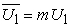(9)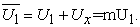(10)

For series controllers, UX can be introduced: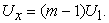(11)

### 4. Power Flow Calculation of DC Grid with Power Flow Controller

4.1. Power Flow Control Analysis

Combined with the second chapter of this article, the power flow calculation method of the DC power grid is derived. The third chapter analyzes the external characteristics of the two power flow controllers. This chapter mainly analyzes the influence of the controller on the DC power grid and brings it into the power flow calculation. Deduce the power flow calculation of the DC grid with power flow controller.

4.1.1. Influence of Variable Resistance Type Power Flow Controller

According to the external characteristics introduced above, after the controller is installed in the circuit, the resistance of the circuit changes. In the power flow calculation, the nodal admittance matrix is changed. Node admittance matrix after being changed: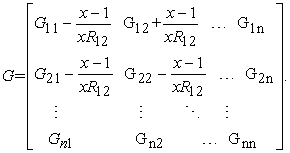(12)

This equivalent method only needs to change the line parameters in the original power flow calculation.

4.1.2. Influence of Variable Voltage Type Power Flow Controller

From Figure 6, we can see that after adding a variable voltage controller, the voltage of the line becomes m times the original, and the power equation of the line becomes: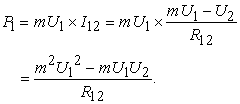(13)

Deriving this formula to the general situation, the output power equation of the nodes at both ends to the line is: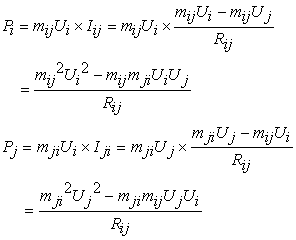(14)

When using Newton Raphson method for power flow calculation, after adding a variable voltage power flow controller, the calculation of the Jacobian matrix is also affected by the controller. The calculation formula after the change: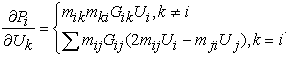(15)

The analysis shows that what this controller changes is the power equation in the power flow calculation.

4.2. Original Power Flow Calculation

The example topology used in this article is shown in Figure 7. It is a three-terminal three-wire DC power grid, the line parameters are shown in Table 1.

The converter station 3 is selected as the voltage station (V node) with a voltage of 125V (the inter-stage voltage is 250kV), and the remaining two stations are set as constant active power stations (P nodes). Suppose the input power of each station is 200 MW and 100 MW respectively. Enter the parameters into the program and execute the load flow calculation to obtain the results. As shown in Table 2 and Table 3.

4.3. Power Flow Calculation of DC Grid with Power Flow Controller
4.3.1. Power Flow Calculation with Variable Resistance Type Power Flow Controller

In the DC power grid shown in Figure 7, the power flow controller is installed on the converter stations 1 and 2, and the resistance of the series controller is RX=1Ω. The topology after adding the controller is shown in Figure 8. According to formula (8), the controller influence value can be calculated as 1.28. According to the above, the influence is equivalent to the power flow calculation, the conductance parameters of the original system are changed, and the power flow distribution is calculated. Then build a model in the power flow calculation software for calculation. The two calculation results are shown in Table 4 and Table 5.

• Figure 8. Grid structure with load flow controller

It can be seen from the table that the error of the node voltage calculated by this method and the PSAT algorithm does not exceed 0.1%, and the line power error is approximately equal to 0.01%, which proves the rationality of the algorithm proposed in this paper.

4.3.2. Power Flow Calculation with Variable Voltage Type Power Flow Controller

Replace the power flow controller in Figure 8 with a variable voltage type, the influence of the controller becomes the change of the voltage on the line, set the transformation ratio to m=1.010, if it is a series controller, from equation (11) The voltage reference value UX is calculated, the influence is equivalent to the power flow calculation, and the power flow distribution is calculated. Then build a model in the power flow calculation software for calculation. The two calculation results are shown in Table 6 and Table 7:

It can be seen from the table that the error of the node voltage calculated by this method and the PSAT algorithm does not exceed 0.1%, and the line power error is not exceed 0.01%, which proves the rationality of the algorithm proposed in this paper.

### 5. Conclusion

Starting from the power flow calculation, this paper analyzes the working principle of the DC power flow controller, and studies the power flow calculation after adding the power flow controller. The main conclusions of the work are as follows:

(1) Based on the power flow calculation of the AC power grid, according to the characteristics of the DC power grid, the power flow calculation method suitable for the DC power grid, the Newton-Raphson method and the fast power flow algorithm are analogized. And deduced the optimal power flow algorithm.

(2) Through the understanding of existing DC power flow controllers, this article introduces two kinds of power flow controllers, variable voltage type and variable resistance type. Analyze the topological structure of the two controllers and their influence on the DC grid, and reflect this influence into the power flow calculation. A three-terminal DC grid is used to verify the correctness of the proposed method.

### References

  Wen Jialiang, Wu Rui, Peng Chang, et al. Analysis of DC grid prospects in China[J]. Proceedings of the CSEE, 2012, 32(13): 7-12. In article  Li Guoqing, Bian Jing, Wang He, Yang Yang, Wang Haoxiang. Overview of DC power flow analysis and control research [J]. High Voltage Technology, 2017, 43(04): 1067-1078. In article  Liu Yun. Research on the Construction and Optimization of Complex DC Grid [D]. North China Electric Power University (Beijing), 2016. In article  Wang Yu. DC grid control method and simulation research [D]. China Electric Power Research Institute, 2013. In article  Meyer C, Höling M, Peterson A, et al. Control and design of DC grids for offshore wind farms[J]. IEEE Trans, on Industry Applications, 2007, 43(6): 1475-1482. In article View Article  Jovcic D, Hajian M, Zhang H, et al. Power flow control in DC transmission grids using mechanical and semiconductor based DC/DC devices[C]//10th IET International Conference on AC and DC Power Transmission. 2012: 1-6 In article View Article  Qing Mu, Jun Liang, Yalou Li, et al. Power flow control devices in DC grids[C]//2012 IEEE Power and Energy Society General Meeting. San Diego, CA: IEEE, 2012: 1-7. In article View Article  Veilleux E, Boon-TeckOoi. Multiterminal HVDC with thyristor power-flow controller[J]．IEEE Transactions on Power Delivery, 2012, 27(3): 1205-1212. In article View Article  Haileselassie T M, Uhlen K. Power flow analysis of multi-terminal HVDC networks[C]//2011 IEEE Trondheim Power Tech. Trondheim, Norway: IEEE, 2011: 1-6. In article View Article  Tinney W F, Hart C E. Power flow solution by Newton’s method[J]. IEEE Transactions on Power Apparatus and Systems, 1967, PAS-86(11): 1449-1460. In article View Article  Wood A J, Wollenberg B F. Power generation, operation and control[M]. 2nd edition. New York NY, USA: Wiley, 1996. In article View Article  Tinny W F, Hart C E. Power flow solution by Newton’s method[J]. IEEE Transactions on Power Apparatus and Systems, 1967, PAS-86(11): 1449-1460. In article View Article  Qing Mu, Jun Liang, Yalou Li, et al. Power flow control devices in DC grids[C]//2012 IEEE Power and Energy Society General Meeting. San Diego, CA: IEEE, 2012: 1-7. In article View Article  Xu Feng, Xu Zheng. A modular multi-level power flow controller suitable for multi-terminal DC systems[J]. Automation of Electric Power Systems, 2015, 39(03): 95-102. In article

Published with license by Science and Education Publishing, Copyright © 2020 Xunyu Liu, Xiaotian Xu, Yu Qi Pang and Xinyuan ZhangThis work is licensed under a Creative Commons Attribution 4.0 International License. To view a copy of this license, visit http://creativecommons.org/licenses/by/4.0/